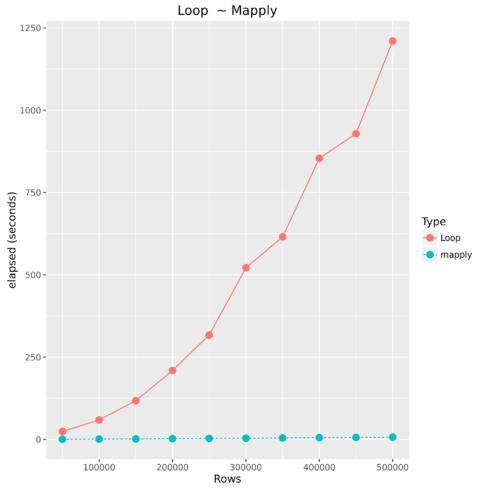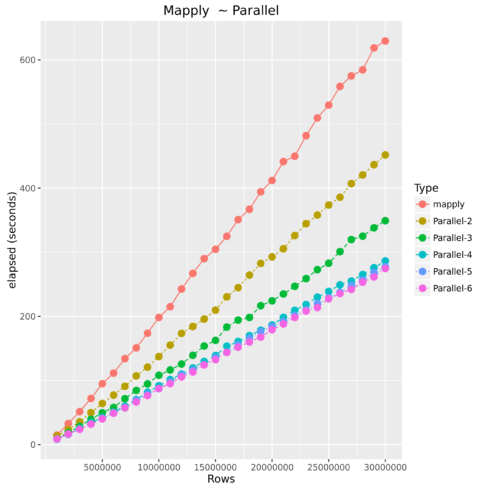Want to share your content on R-bloggers? click here if you have a blog, or here if you don't.

After using R for a few years people come to realize how inefficient loops are (in all languages), even after pre-allocating memory. Then you begin to use the apply functions and revel at their speed. However, once you have datasets > 10-15 million the apply family can begin to creep along (relative to the amount of logic in your function). Luckily, most datasets I work with are between 1 – 5 million records and do not encounter performance issues too often. However, when we do run into large datasets we have our parallel packages.

## Demo Machine

While writing this demo from my home laptop I was not seeing performance gains in parallel, because I was running out of RAM to maximize the efficiency of parallel processing. Therefore, I decided to checkout Google’s Cloud and was quite happy with the aesthetics and performance of their platform. Now, I do not work for Google, but it is worth checking out their trial.

custom (8 vCPUs, 32 GB memory)

## For Loop

Most programmers know to try and avoid loops whenever possible because of they are extremely resource intensive. However, with languages such as R, the majority of the time spent is manipulating the dataset. Oftentimes this includes cleaning and feature engineering which requires user-defined functions. If you have less than 10,000 observations and the function is not overly complex then for loops are fine. The example below processes 150 observations in three hundredth of a second.

```IrisClassifier  <- function(df,i){
if(df[i,] >= 5.8 & df[i,] >= 1.8){
return('virginica')
}else if(df[i,] <= 5.7 & df[i,] <= 1){
return('setosa')
}else{
return('versicolor')
}
}
iris\$Check <- ''
system.time(
for(i in 1:nrow(iris)){
iris\$Check[i] <- IrisClassifier(iris,i)
}
)
```
User Time System Time Elapsed Time
0.03 0.00 0.03

### Vectorized Operations

How does the apply family speed up our functions? It converts a scalar (single item) process to a vector (array) process. Think of a mill having to cut lumber piece-by-piece or having one large saw and applying it to all of the lumber at once.

```IrisClassifier  <- function(col1,col2){
if(col1 >= 5.8 & col2 >= 1.8){
return('virginica')
}else if(col1 <= 5.7 & col2 <= 1){
return('setosa')
}else{
return('versicolor')
}
}

system.time(iris\$Check <- mapply(psuedoClassifier,iris[,1],iris[,4]))
```
User Time System Time Elapsed Time
0.00 0.00 0.00

## Loop vs. mapply

Required Libraries:

```library(doSNOW)
library(foreach)
library(data.table)
library(sqldf)
library(ggplot2)
```

### The Dataset

For this example we will be using a simple dataset starting with 1,000,000 million observations and increment by 1,000,000 to 30,000,000 million observations. The entire script is located at the end of this example.

```df <-   df <- data.frame(a = round(rnorm(values[i],mean = 18,sd = 10),0),
b = round(rnorm(values[i],mean = 10,sd = 11),0),
c = round(rnorm(values[i],mean = 400,sd = 44),0),
d = round(rnorm(values[i],mean = 100,sd = 33),0),
e = round(rnorm(values[i],mean = 11,sd = 3),0),
f = round(rnorm(values[i],mean = 223,sd = 1000),0)
)
```

### Test Function

For this example we will use the `mapply` to show how to apply a function with multiple parameters. I find most articles online only demonstrating how to apply with one parameter so I used this as an excuse to demonstrate applying a function with multiple parameters.

```unspirited.func <- function(a,b,c,d,e,f){
if(a > 20 & b  300 & c  30 & log(c)> 6) {
return('Fail - A')
}else if(b > 38 & log(c)> 6) {
return('Fail- B')
}else if(d > 175 & log(c)> 6) {
return('Fail - D')
}else if(e > 18 & log(c)> 6) {
return('Fail - E')
}else if(f > 1989 & log(c)> 6) {
return('Fail - F')
}else{
return('Unsure')
}
}
```

### Comparing For Loop to Vectorized OperationsType Number of Observations Elapsed Time
Loop 50000 24.593
mapply 50000 0.536
Loop 100000 59.314
mapply 100000 1.228
Loop 150000 117.658
mapply 150000 1.865
Loop 200000 209.694
mapply 200000 2.658
Loop 250000 317.212
mapply 250000 3.283
Loop 300000 521.578
mapply 300000 3.816
Loop 350000 615.212
mapply 350000 4.625
Loop 400000 854.06
mapply 400000 5.571
Loop 450000 928.809
mapply 450000 6.437
Loop 500000 1210.446
mapply 500000 7.179

## Mapply vs. Parallel Mapply

Parallel Operations
Luckily most current laptops, pcs, and cloud machines all offer multiple cores of processing power. Therefore, how does parallel processing work? Essentially, R starts up n number of instances and sends subsets of the original data to be processed in those instances using its own processing core, and then finally returning the results back together.Type Number of Observations Elapsed Time
mapply 1000000 14.851
Parallel-2 1000000 13.641
Parallel-3 1000000 9.407
Parallel-4 1000000 8.741
Parallel-5 1000000 8.326
Parallel-6 1000000 8.977
mapply 5000000 95.012
Parallel-2 5000000 64.179
Parallel-3 5000000 49.724
Parallel-4 5000000 42.357
Parallel-5 5000000 40.959
Parallel-6 5000000 40.204
mapply 10000000 198.36
Parallel-2 10000000 137.413
Parallel-3 10000000 108.221
Parallel-4 10000000 91.929
Parallel-5 10000000 87.314
Parallel-6 10000000 87.57
mapply 15000000 304.673
Parallel-2 15000000 209.789
Parallel-3 15000000 162.592
Parallel-4 15000000 138.99
Parallel-5 15000000 135.785
Parallel-6 15000000 132.459
mapply 20000000 411.984
Parallel-2 20000000 292.82
Parallel-3 20000000 224.262
Parallel-4 20000000 186.6
Parallel-5 20000000 184.216
Parallel-6 20000000 179.269
mapply 25000000 529.586
Parallel-2 25000000 373.688
Parallel-3 25000000 282.885
Parallel-4 25000000 238.923
Parallel-5 25000000 229.101
Parallel-6 25000000 227.555
Parallel-2 30000000 451.788
Parallel-3 30000000 349.257
Parallel-4 30000000 286.526
Parallel-5 30000000 277.594
Parallel-6 30000000 274.735

Considerations

• Required Setup time
• Parallel will end up using more RAM
• Shared server resources

## Final Note

After hearing Max Kuhn speak a few years ago he said always check to see if someone has already written the function you are coding. After, writing this post I did stumble upon some builtin in parallel apply functions. However, I have not researched exactly how these operate.

The Entire Script

```###############################################################################################################################
# Name             :  Parallel Process Example
# Date             :  2016-09-03
# Author           :  Christopher M
# Purpose          :  ~
###############################################################################################################################
# ver    user        date(YYYYMMDD)        change
# 1.0     ~          20160903              initial
###############################################################################################################################

library(parallel)
library(ggplot2)

## Display number of CPU cores on host
detectCores(all.tests = FALSE, logical = TRUE)

## Example function for processing
unspirited.func <- function(a,b,c,d,e,f){

if(a > 20 & b  300 & c  30 & log(c)> 6) {
return('Fail - A')
}else if(b > 38 & log(c)> 6) {
return('Fail- B')
}else if(d > 175 & log(c)> 6) {
return('Fail - D')
}else if(e > 18 & log(c)> 6) {
return('Fail - E')
}else if(f > 1989 & log(c)> 6) {
return('Fail - F')
}else{
return('Unsure')
}

}

## kfold function to split data for parallel processing
kfold <- function(df,k){
require(sqldf)
if( length(which(colnames(df)=='folds'))>0){
stop(print('Rename column(s) named folds'))
break

}
#randomize the data
df<-sqldf("SELECT * FROM df order by random()")

#Create equally size folds
df\$folds <- cut(seq(1,nrow(df)),breaks=k,labels=FALSE)

return(df)

}

## function for parallel processing
parallelApply <- function(df,cols,func,cores){

require(doSNOW)
require(foreach)
require(data.table)
cl <- makeCluster(cores, type="SOCK")
registerDoSNOW(cl)

## Create ID to compute in parallel
df <- kfold(df,cores)

## Process data in parallel
system.time(NewDataFrame <- foreach(i = 1:cores) %dopar% {
tmpdata <- subset(df, folds == i)
mapply(func,tmpdata[,cols],tmpdata[,cols],tmpdata[,cols],tmpdata[,cols],tmpdata[,cols],tmpdata[,cols])

})

## Bind data back into dataframe
NewDataFrame <- rbindlist(Map(as.data.frame, NewDataFrame))
colnames(NewDataFrame) <- c('ParRow')
df <- cbind(df,NewDataFrame)
stopCluster(cl)
return(df)

}

## values for dataframe length
values <- seq(1e6,30e6,1e6)

capture <- data.frame()

for(i in 1:length(values)){
print(paste("processing:",values[i],"rows. There are",length(values)-i,"iterations left to complete"))

df <- data.frame(a = round(rnorm(values[i],mean = 18,sd = 10),0),
b = round(rnorm(values[i],mean = 10,sd = 11),0),
c = round(rnorm(values[i],mean = 400,sd = 44),0),
d = round(rnorm(values[i],mean = 100,sd = 33),0),
e = round(rnorm(values[i],mean = 11,sd = 3),0),
f = round(rnorm(values[i],mean = 223,sd = 1000),0)
)
##______________________________________________________________________________
## Apply

performance <- system.time(df\$Check <- mapply(unspirited.func,df[,1],df[,2],df[,3],df[,4],df[,5],df[,6]))
capture <- rbind(capture,data.frame(Type = 'mapply',Rows = values[i],elapsed = performance,PercentDifference = 1))
df\$Check <- NULL
print('finished')
print(capture)
##______________________________________________________________________________
## Parallel Apply
base <- as.numeric(subset(capture,Rows == values[i] & Type == 'mapply'))
for(x in 2:6){
print(paste('running on',x,'cores'))
performance <- system.time(df <- parallelApply(df,c(1,2,3,4,5,6),unspirited.func,x))
capture <- rbind(capture,data.frame(Type = paste('Parallel-',x,sep=''),Rows = values[i],elapsed = performance,
PercentDifference = abs(1-(performance/base))))
df\$ParRow <- NULL
df\$folds <- NULL
}
gc()
print(capture)
}
options(scipen=999)

## Example plots
ggplot(subset(capture,Type != 'Loop'),aes(Rows,elapsed,color = Type))+
geom_line( aes(linetype=Type))+
geom_point(size=3 )+
ylab("elapsed (seconds)") +
geom_text(data=subset(capture,Rows ==max(capture\$Rows)),
aes(label=Type))+
ggtitle("Mapply  ~ Parallel")

tmp <- subset(capture,Type != 'mapply')
tmp\$PercentDifference <- tmp\$PercentDifference + 1
mapply <- subset(capture,Type == 'mapply')
capture_ <- rbind(tmp,mapply)

ggplot(subset(capture_,Type != 'Loop'),aes(Rows,PercentDifference,color = Type))+
geom_line( aes(linetype=Type))+
geom_point(size=3 )+
ylab("Performance Increase") +
ggtitle("Mapply  ~ Parallel") +
scale_x_continuous(breaks = seq(5e6,30e6,5e6))+
scale_y_continuous(breaks = seq(0,3,.1))
```

Related Post